# How to use Google Sheets ARRAYFORMULAWritten by
Holly MeikleAlthough Google Sheets offers a variety of intelligent functions that help streamline complex calculations within our spreadsheets, it can become a tedious process to insert and apply functions to each cell or range of data. If you’re looking to simplify and streamline the process of applying functions to multiple cells or ranges of data, then you need to learn how to use the Google Sheets ARRAYFORMULA.

The ARRAYFORMULA is an array function that outputs the values from a cell range into multiple rows and columns. Unlike a standard formula, it’s expandable, so it will work on any new data as soon as it’s added. Moreover, it can be used in conjunction with non-array formulae, including SUMIF, VLOOKUP, or FILTER. Essentially, the ARRAYFORMULA will allow you to return an array of values using just one single formula.

Let’s explore in more detail what the ARRAYFORMULA is, why you would need to use it, and how you can use it effectively in Google Sheets.

## What is the ARRAYFORMULA?

The ARRAYFORMULA is an expandable array function. This means that you only need to insert the function into one cell, and then the function is automatically applied to the subsequent rows throughout the length of a whole column.

As a result, you can return multiple values from a range of cells with just one ARRAYFORMULA, rather than returning a single value from a non-array formula.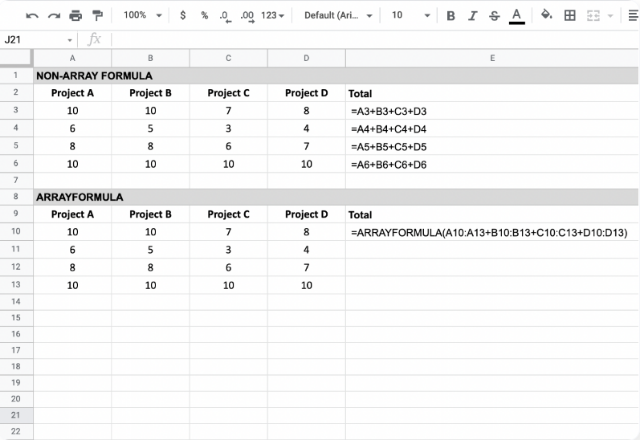Let’s take a look at the syntax, so you can better understand how it works and how to use it combined with other functions in Google Sheets.

### Syntax

The syntax for the ARRAYFORMULA is as follows:

=ARRAYFORMULA(array_formula)
array_formula – this parameter can either be

• a range
• a mathematical expression using one cell range or multiple ranges of the same size
• a function that returns a result greater than one cell
You can also add the ARRAYFORMULA to an existing non-array function in your spreadsheet too. Simply pressing the keyboard shortcut Ctrl + Shift + Enter, and Google Sheets will automatically add ARRAYFORMULA() around your function.

As you can see, the ARRAYFORMULA syntax is a lot more simple than most people think. Let’s explore in more detail why the ARRAYFORMULA is the best option when applying functions to multiple cells in your spreadsheet.

### Why use ARRAYFORMULA in Google Sheets?

As well as saving time and manual effort by offering an alternative to manual calculations, the ARRAYFORMULA also offers other benefits.

Let’s take a look at just a few:

• Batch size calculations: Instead of having to use similar functions that calculate values individually, you can use one single formula to your whole dataset, regardless of the size. This avoids the risk of heavy spreadsheets that contain multiple formulas in a single calculation.
• Flexible: A single change or modification to any cells will automatically affect the value of the ARRAYFORMULA calculation. This means that you can easily edit cell values, and any values relying on these cells will automatically adjust accordingly.
• Dynamic: When you add a new row within your cell range, the ARRAYFORMULA will automatically be applied to it, so you don’t have to manually input another function.
Although Google Sheets may offer “Suggested autofill” to apply the function to the rest of your rows, the autofill will not be able to provide the same benefits as above. For example, If you alter your values, there is the risk that the autofill will not be able to update all of your cell data correctly. This would leave you with out-of-date, inaccurate information.

Now that you understand the many benefits of using the ARRAYFORMULA in mass calculations, Let’s explore how to use the Google Sheets ARRAYFORMULA in the following examples.

### How to use Google Sheets ARRAYFORMULA

Let’s say I’m a teacher and have a dataset with a list of students and results obtained in different projects. I want to calculate the total points per project to then obtain the average.

Usually, I would add up the cell values individually, as shown below: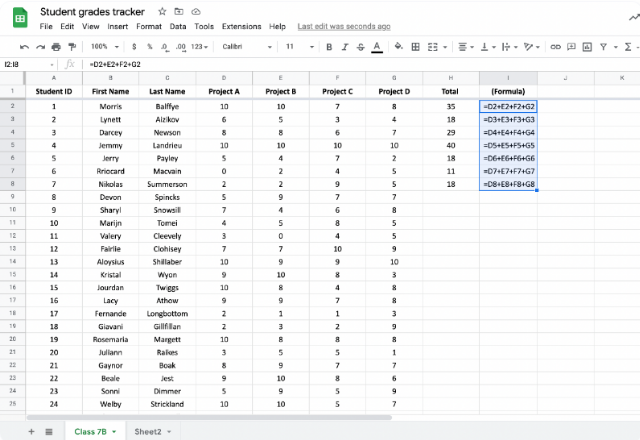However, this method would soon be problematic in two recurrent circumstances:
• Increase in data and formulae: If I decide to increase the size of data or use more formulae, my spreadsheet wouldn’t run as smoothly.
• Regular updates: Any modification to my data means having to change every formula used in it.
By using the ARRAYFORMULA, I can quickly add up results for all students, regardless of the dataset size or the number of formulae used. I can add, remove, or edit content as I wish, and the formula will apply to the data automatically.

#### Example 1: Basic ARRAYFORMULA

Building on the previous example, in order to calculate the totals, you can use the ARRAYFORMULA as shown below. Notice that, unlike before where I added individual cells, I am now adding the ranges.

The ARRAYFORMULA should look something like this:

=ARRAYFORMULA(D2:D+E2:E+F2:E+G2:G)
In order for the ARRAYFORMULA to function properly, make sure the cells don’t contain any values or functions.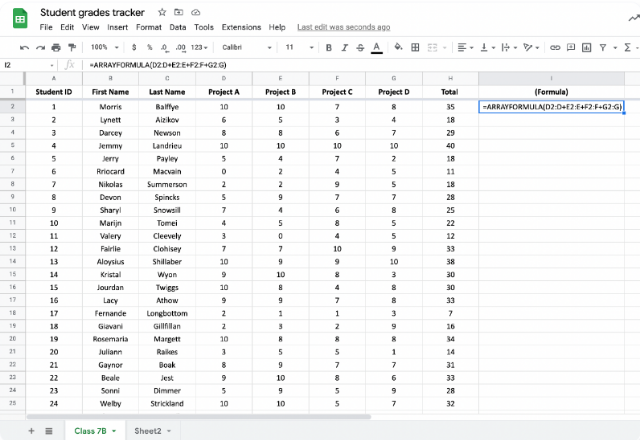Please note: By ending the range of each column with the letter, (D2:D) you can add the entire column as the array. This means that if you add any new rows of data in the columns, the ARRAYFORMULA function will automatically be applied.

As you can see, using the basic formula is very straightforward! Let’s see how the ARRAYFORMULA combines with other non-array functions in the following examples.

#### Example 2: IF with ARRAYFORMULA

You’ve probably noticed a sequence of zeroes at the end of the column after applying the formula in the previous example. You can avoid this by changing the cell ranges in the function, i.e. D2:D52. However, you can also do this by combining the ARRAYFORMULA with the IF function.

I’ve added an IF statement that tells the function to leave the ‘Total’ column blank if there is no name in column A, by typing:

=ARRAYFORMULA(IF(A2:A<>””,D2:D+E2:E+F2:E+G2:G,””))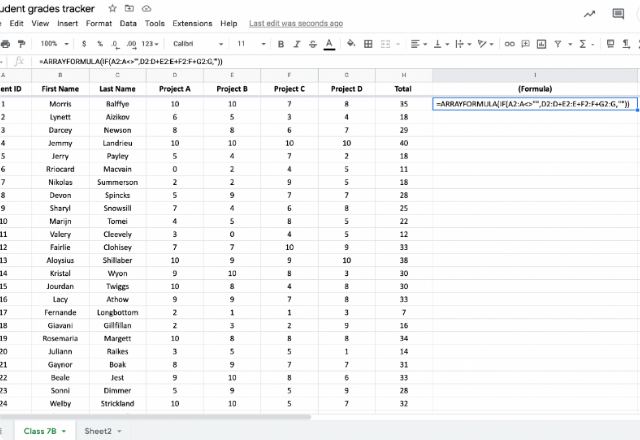Now, there are only blank cells, without zeroes, after the last row of data in column A. Let’s include a row for another student after row 50 to test its expandable nature.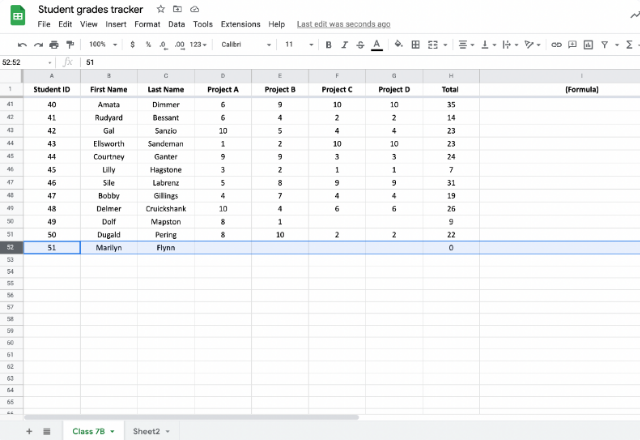As soon as you start typing in content, the last column will automatically include a zero. This is a significant benefit of using ARRAYFORMULA. When you have a dataset that changes often, using ARRAYFORMULA will let you make your changes without having to adjust any of the equations in the spreadsheet.

Let’s take a look at a more advanced alternative to the IF statement, by using SUMIF and SUMIFS formulas.

#### Example 3: SUMIF with ARRAYFORMULA

Let’s say I’ve grouped the students into 3 different groups, and I want to find the group total on ‘Project D’. I can use the ARRAYFORMULA in conjunction with the SUMIF function to get the total from these 3 groups.

The syntax for SUMIF and ARRAYFORMULA is:

=ARRAYFORMULA(SUMIF(range, criteria, sum_range))
For this example, I’d use the formula:
=ARRAYFORMULA(SUMIF(B2:B,I2:I,H2:H))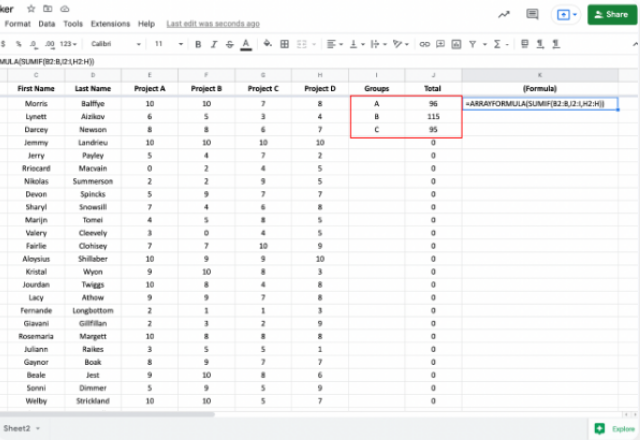As you can see, I now have the total points per group for Project D, as shown in column J. I can use this new output to calculate any other evaluation metric in a quick and efficient way.

As before, zeroes appear sequenced after the last content cell in the ‘Total’ column. Let’s take a look at how combining SUMIFS and ARRAYFORMULA can help quickly fix this, as we did with the IF statement.

For more information on how the SUMIF function works, take a look at this blog post on How to use the SUMIF function in Excel.

### Example 4: VLOOKUP with ARRAYFORMULA

Let’s say I want to look for specific performance data from a specific student. Usually, I could use the VLOOKUP function to do this. However, I would have to input the function multiple times for each criterion, as VLOOKUP only returns a single value. However, when I combine this with the ARRAYFORMULA, I can find multiple values at the same time.

The syntax for VLOOKUP with ARRAYFORMULA is:

=ARRAYFORMULA(VLOOKUP({search-key#1;search-key#2;…}, range, column-index,[sorted/not-sorted])
In this example, I want to find the first name, last name and results for project A and B of student number 10. With ‘Student ID’ as the key, I would use the following formula:
=ARRAYFORMULA(VLOOKUP(\$A\$11,\$A\$1:\$I\$52,{1,3,4,5,6},FALSE))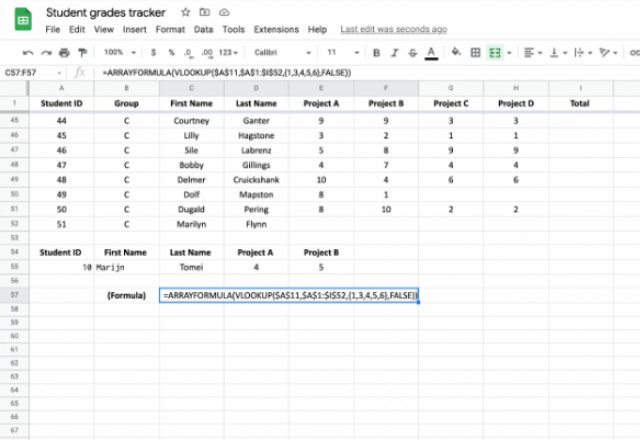#### Example 5: FILTER with ARRAYFORMULA

One of the most common uses when combining FILTER and ARRAYFORMULA is to identify duplicates. In order for it to function, you need to incorporate two basic formulas: COUNTIF and UNIQUE.

Let’s say I want to find duplicate content for student last names to search for possible relatives. I would need to type in the following formula:

=FILTER(UNIQUE(D2:D),ARRAYFORMULA(COUNTIF(D2:D,UNIQUE(D2:D))>1))
Any duplicate values should appear in the cell.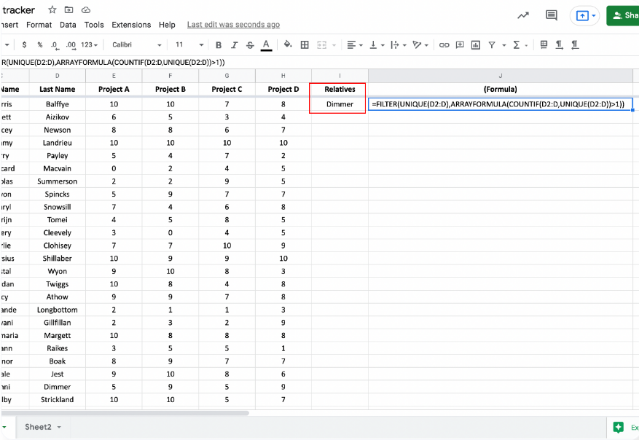As you can see,‘Dimmer’ has appeared in the cell. This means that the formula found more than one cell in the ‘Last Name’ column containing the name Dimmer. This means that I have two students with that same last name.

You can also use this function to help find and remove duplicate data. To find out more about how these functions work individually, read these blog posts on the UNIQUE, COUNTIF and FILTER functions.

#### Example 6: Horizontal arrays

So far, the examples that I’ve shown have all been applied to vertical arrays, that follow a top-down process to calculate a value. The ARRAYFORMULA can also be used just as easily for horizontal arrays, which allows you to use it no matter the structure of your data.

Let’s calculate the ‘Total’ for all projects for each student, but in a horizontal format. I can do this by inputting the following formula:

=ARRAYFORMULA(B5:5+B6:6+B7:7+B8:8).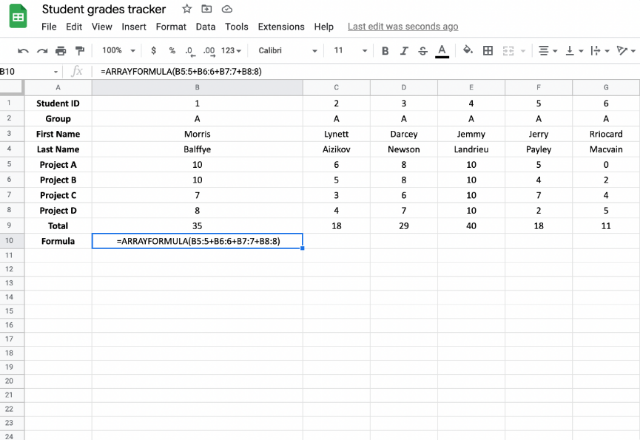Instead of telling the function to go down the list (e.g., from A2:A, which means starting at A2 and going down through column A), I’ve told it to go across the data (e.g., from B5:5, which means starting at B5 and going across through row 5).

By making this simple change, the ARRAYFORMULA is now working horizontally. And just like in the previous example, to get rid of the zeros in the blank columns, I can add an IF statement to the function:

=ARRAYFORMULA(IF(B1:1<>””, B5:5+B6:6+B7:7+B8:8,””))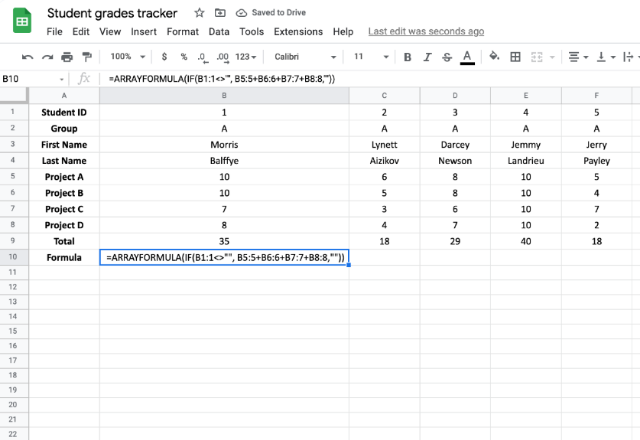#### Example 7: Multidimensional Arrays

You’ve now seen the ARRAYFORMULA used in both vertical and horizontal orientations, but what about a table that includes both of these array types? You can use the ARRAYFORMULA for these tables too!

Here, I’ve created a multiplication table that multiples column A by row 1, by simply typing the following:

=ARRAYFORMULA(B1:M1*A2:A13)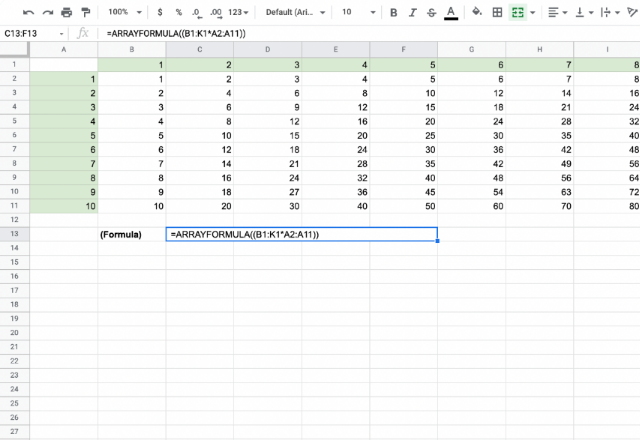#### Example 9: Using ARRAYFORMULA to combine columns

The ARRAYFORMULA not only helps to calculate numeric data, but it can also help to combine text from different columns.

Let’s say I want to combine the ‘First Name’ and ‘Last Name’ columns to create a ‘Full Name’ column. I’ve inserted a column for ‘Full Name’ to include the following formula:

=ARRAYFORMULA(C2:C52&” “&D2:D52)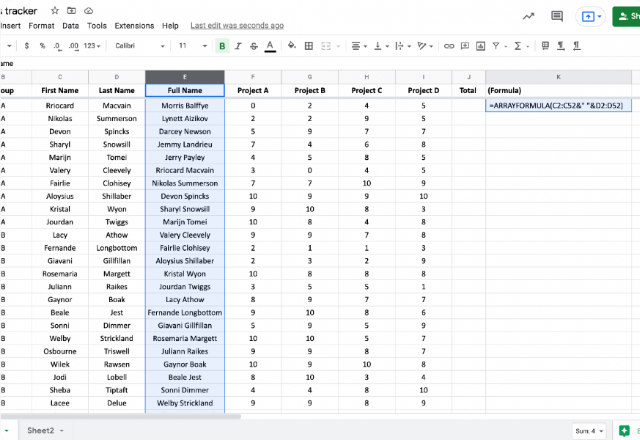As you can see, I have obtained values from two different columns and combined them into one without the need to do manual work.

Please note: This formula will only work if the two arrays are the same size (e.g.‘C2:C52&” “&D2:D52’). If not, Google Sheets won’t be able to perform the calculation.

### How do I create an ARRAYFORMULA in Google Sheets?

And there you have it! The ARRAYFORMULA is an extremely powerful, versatile tool that can help significantly streamline the management of your spreadsheet data. Not only can you return multiple values with just a single function, but you can also combine this with other functions such as IF, VLOOKUP, and SUMIF in order to generate advanced calculations. Mastering the ARRAYFORMULA function in Google Sheets will allow you to work on any data, regardless of the size or type, including numbers and text.

Are you looking to constrain an array result to a specific size? Learn How to use the ARRAY_CONSTRAIN formula in Google Sheets.

Alternatively, check out related blog posts below.

Editor’s note: This is a revised version of a previous post that has been updated for accuracy and comprehensiveness.